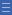## Featured resource

Home > Topdrawer > Fractions > Big ideas

# Big ideas

Big ideas

What is a fraction?

Think beyond your immediate response. Are there other ways fractions are used in mathematics?

Most people would explain a fraction as a way of expressing 'parts of a whole'. While the part-whole idea describes a common use of fractions, it is not the only meaning. The part-whole description does not explain a fraction as a number positioned on a number line, or the use of fractions to express division.

All fractions can be explained as expressing mathematical relationships between quantities. These quantities may be discrete (countable items) or continuous (like area and length). Modelling these various quantities leads to a range of representations for fractions — for example, area diagrams, number lines and discrete objects.

Five different constructs for fractions have been identified: part-whole, measure, division, operator and ratio.

All five of the fraction constructs are included in the Australian Curriculum: Mathematics (2012).

• Years 1 and 2 focus on the part-whole construct, using parts of shapes and parts of groups of objects.
• In year 3, fractions as a measure are introduced, using folded paper strips and number lines.
• Year 6 tackles the use of fractions as operators and fractions expressing division.
• Ratio and rate are introduced in year 7.

Researchers generally agree that it is important for students to develop flexibility in interpreting and using fractions in a range of mathematical contexts. A comprehensive understanding of fractions is needed for proportional reasoning, algebra and probability.

Further information can be found in the article One-to-One Student Interviews Provide Powerful Insights and Clear Focus for the Teaching of Fractions in the Middle Years available on the AAMT website.

Yes

Yes

Name Class SectionPart-whole fractions Folder 17Fractions as a measure Folder 17Fractions as division Folder 17Fractions as operators Folder 17Fractions as ratios Folder 17